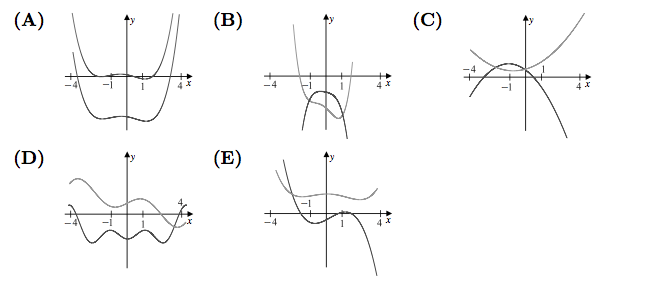###### back to index | new

Given the function $f(x) = 2x^2 - 3x + 7$ with domain {$-2, -1, 3, 4$}, what is the largest integer in the range of $f$?

The domain of the function $f(x)=\log_{\frac12}(\log_4(\log_{\frac14}(\log_{16}(\log_{\frac1{16}}x))))$ is an interval of length $\tfrac mn$, where $m$ and $n$ are relatively prime positive integers. What is $m+n$?

Suppose that $f(x+3)=3x^2 + 7x + 4$ and $f(x)=ax^2 + bx + c$. What is $a+b+c$?

A function $f$ has domain $[0,2]$ and range $[0,1]$. (The notation $[a,b]$ denotes $\{x:a \le x \le b \}$.) What are the domain and range, respectively, of the function $g$ defined by $g(x)=1-f(x+1)$?

Let $a > 0$, and let $P(x)$ be a polynomial with integer coefficients such that $P(1) = P(3) = P(5) = P(7) = a$, and $P(2) = P(4) = P(6) = P(8) = -a$. What is the smallest possible value of $a$?

Find all polynomials $f(x)$ such that $f(x^2) = f(x)f(x+1)$.

Let $x, y \in [-\frac{\pi}{4}, \frac{\pi}{4}], a \in \mathbb{Z}^+$, and $$\left\{ \begin{array}{rl} x^3 + \sin x - 2a &= 0 \\ 4y^3 +\frac{1}{2}\sin 2y +a &=0 \end{array} \right.$$ Compute the value of $\cos(x+2y)$

Find the range of the function $$y=x+\sqrt{x^2 -3x+2}$$

Which pair contains same functions: (A) $f(x)=\sqrt{(x-1)^2}$ and $g(x)=x-1$ (B) $f(x)=\sqrt{x^2 -1}$ and $g(x)=\sqrt{x+1}\cdot\sqrt{x-1}$ (C) $f(x)=(\sqrt{x -1})^2$ and $g(x)=\sqrt{(x-1)^2}$ (D) $f(x)\displaystyle\sqrt{\frac{x^2-1}{x+1}}$ and $g(x)=\displaystyle\frac{\sqrt{x^2-1}}{\sqrt{x+1}}$ Select all correct answers.

Let $a$ and $k$ be two positive integers, and function $f(x)=3x+1$. If $f(x)$'s domain is $\{1, 2, 3, k\}$ and range is $\{4, 7, a^4, a^2 + 3a\}$, find the value of $a$ and $k$.

Which of the following function is the same as $y=\sqrt{-2x^3}$? (A) $y=x\sqrt{-2x}\qquad$ (B) $y=-x\sqrt{-2x}\qquad$ (C) $y=-\sqrt{2x^3}\qquad$ (D) $y=x^2\sqrt{-2/x}$

Let $f(x)$ be an odd function and $g(x)$ be an even function. If $f(x)+g(x)=\frac{1}{x-1}$, find $f(x)$ and $g(x)$.

If $f\Big(\displaystyle\frac{x+1}{x}\Big)=\displaystyle\frac{x^2+x+1}{x^2}$, find $f(x)$.

If $f(x)$ is an odd function defined on $\mathbb{R}$, compute $f(0)$.

Let $f(x)= \sqrt{ax^2+bx}$. For how many real values of $a$ is there at least one positive value of $b$ for which the domain of $f$ and the range of $f$ are the same set?

The nonzero coefficients of a polynomial $P$ with real coefficients are all replaced by their mean to form a polynomial $Q$. Which of the following could be a graph of $y = P(x)$ and $y = Q(x)$ over the interval $-4\leq x \leq 4$?The graph of the function $f$ is shown below. How many solutions does the equation $f(f(x))=6$ have?

Determine all polynomials such that $P(0) = 0$ and $P(x^2 + 1) = P(x)^2 + 1$.

A binary operation $\diamondsuit$ has the properties that $a\,\diamondsuit\, (b\,\diamondsuit \,c) = (a\,\diamondsuit \,b)\cdot c$ and that $a\,\diamondsuit \,a=1$ for all nonzero real numbers $a, b,$ and $c$. (Here $\cdot$ represents multiplication). The solution to the equation $2016 \,\diamondsuit\, (6\,\diamondsuit\, x)=100$ can be written as $\tfrac{p}{q}$, where $p$ and $q$ are relatively prime positive integers. What is $p+q?$

Let $f(x)=x^{-\frac{k^2}{2}+\frac{3}{2}k+1}$ be an odd function where $k$ is an integer. If $f(x)$ is monotonically increasing when $x\in(0,+\infty)$, find all the possible values of $k$.

Let $P(x)$ be a nonzero polynomial such that $(x-1)P(x+1)=(x+2)P(x)$ for every real $x$, and $(P(2))^2 = P(3)$. Then $P\big(\frac72\big)=\tfrac{m}{n}$, where $m$ and $n$ are relatively prime positive integers. Find $m + n$.

Find all functions $f:\mathbb{R}\rightarrow\mathbb{R}$ such that $$x^2f(x)+f(1-x)=2x-x^4$$

Find all functions $f:\mathbb{Q}\rightarrow\mathbb{Q}$ such that the Cauchy equation $$f(x+y)=f(x)+f(y)$$ holds for all $x, q\in\mathbb{Q}$.

Find the range of function $y=x+\sqrt{x^2 -3x+2}$.

Solve $$\Big|\frac{1}{\log_{\frac{1}{2}}x+2}\Big|> \frac{3}{2}$$